# Telangana SSC Board (TS SSC) Question Paper 2 for Class 10th Maths 2017 In PDF

## Telangana 10th Annual Exam Question Papers 2017 Maths Paper 2 with Solutions – Free Download

Telangana SSC Class 10 Maths 2017 Question Paper 2 with solutions are available at BYJU’S have stepwise solutions, so that students can understand them easily. Students can access solutions for Telangana 10th Annual Exam Question Papers 2017 Maths Paper 2 at BYJU’S to verify their results after practising them. Also, several previous year question papers of Telangana Class 10 Maths are given here, which will boost the preparation of students for the exam. Question Papers will help the students in analysing the exam pattern and they can experience different ways of asking questions of Maths in the board examination. This way of preparation will help the students to excel their analytical skills.

### Telangana Board SSC Class 10 Maths 2017 Question Paper 2 with Solutions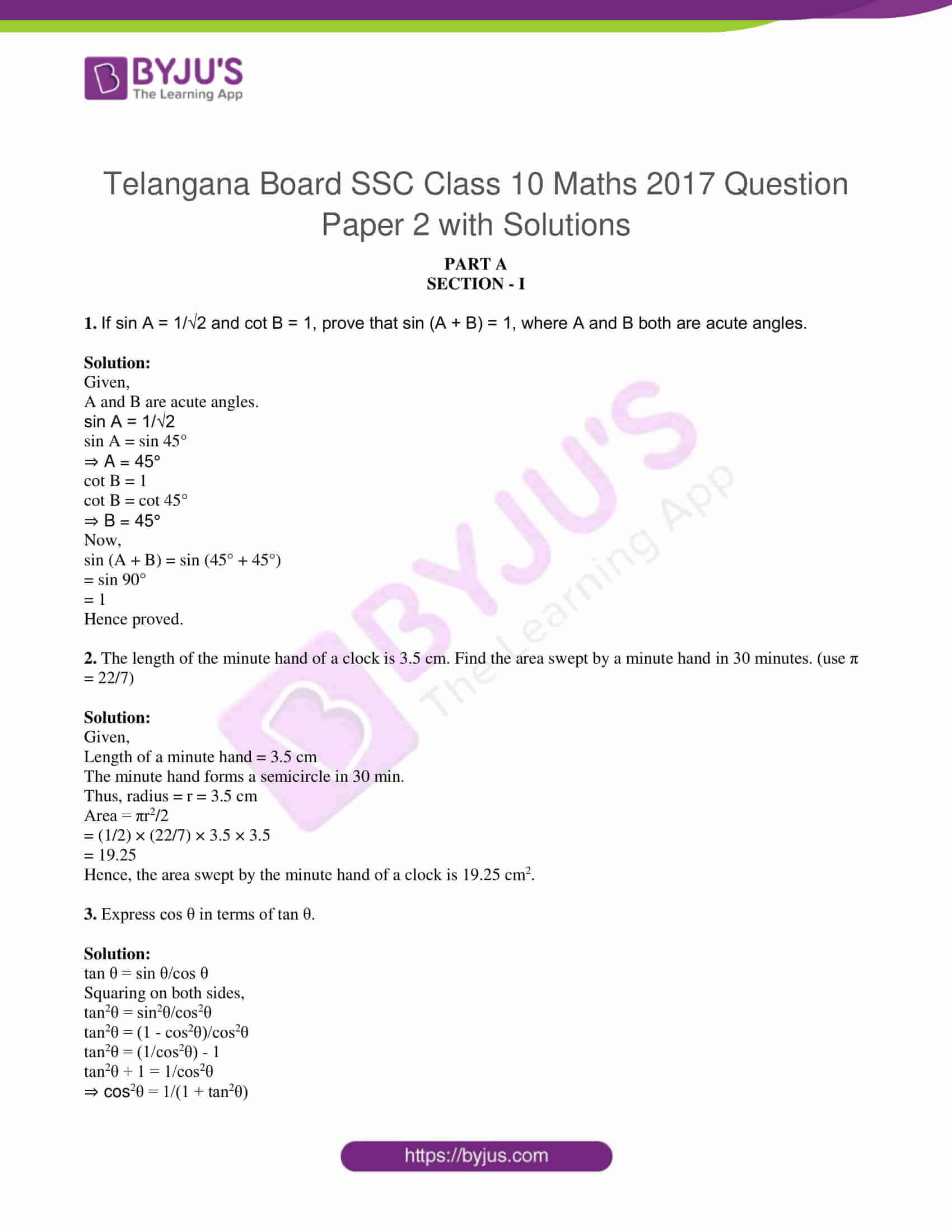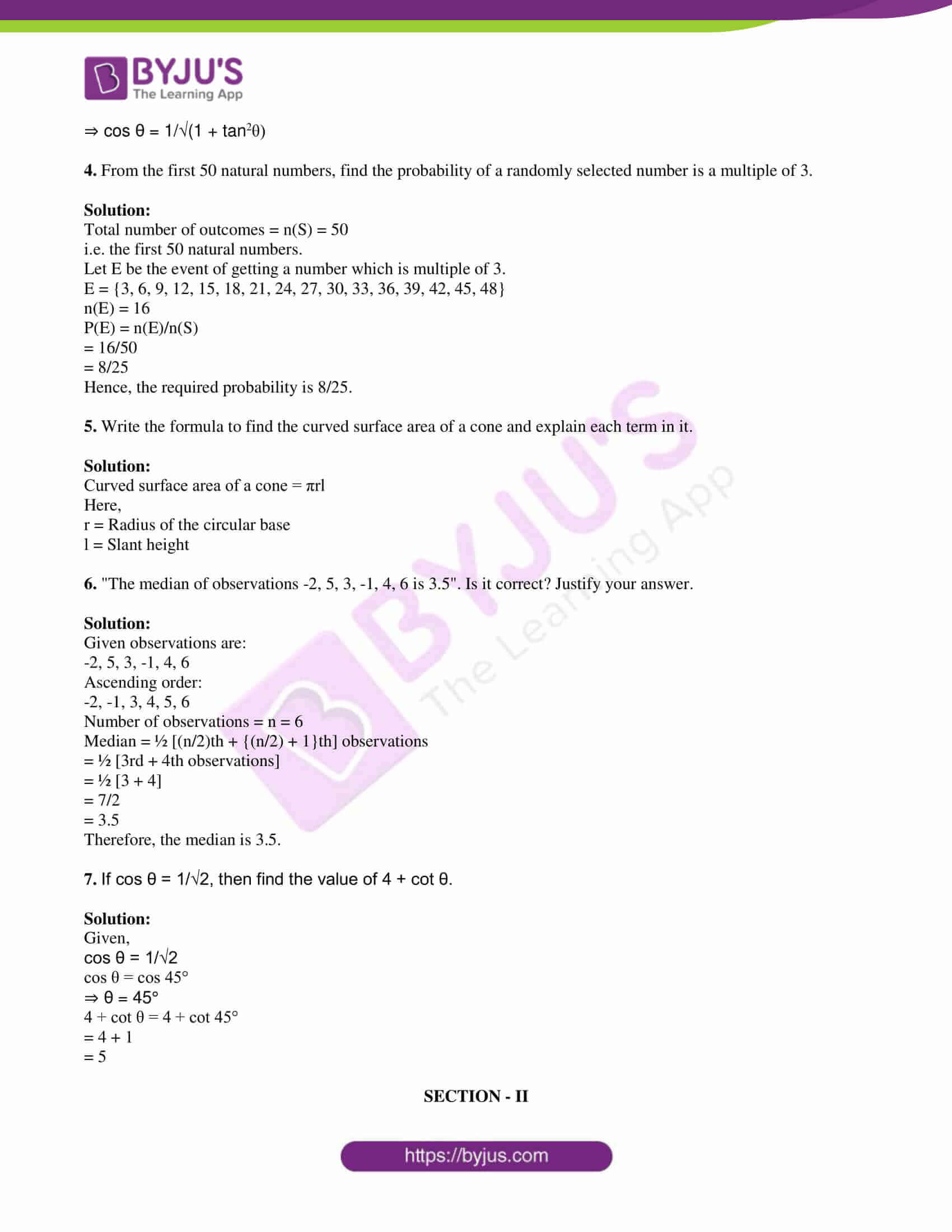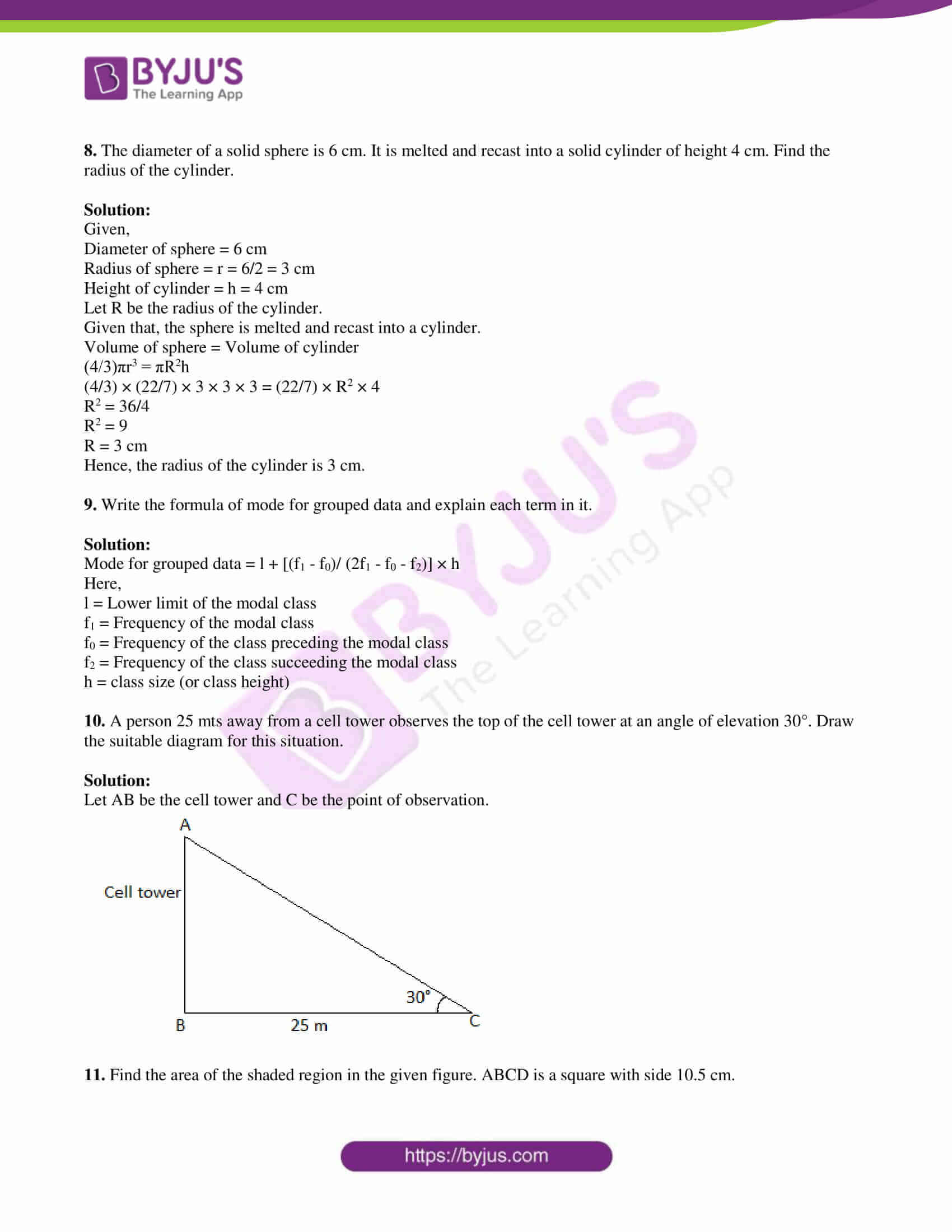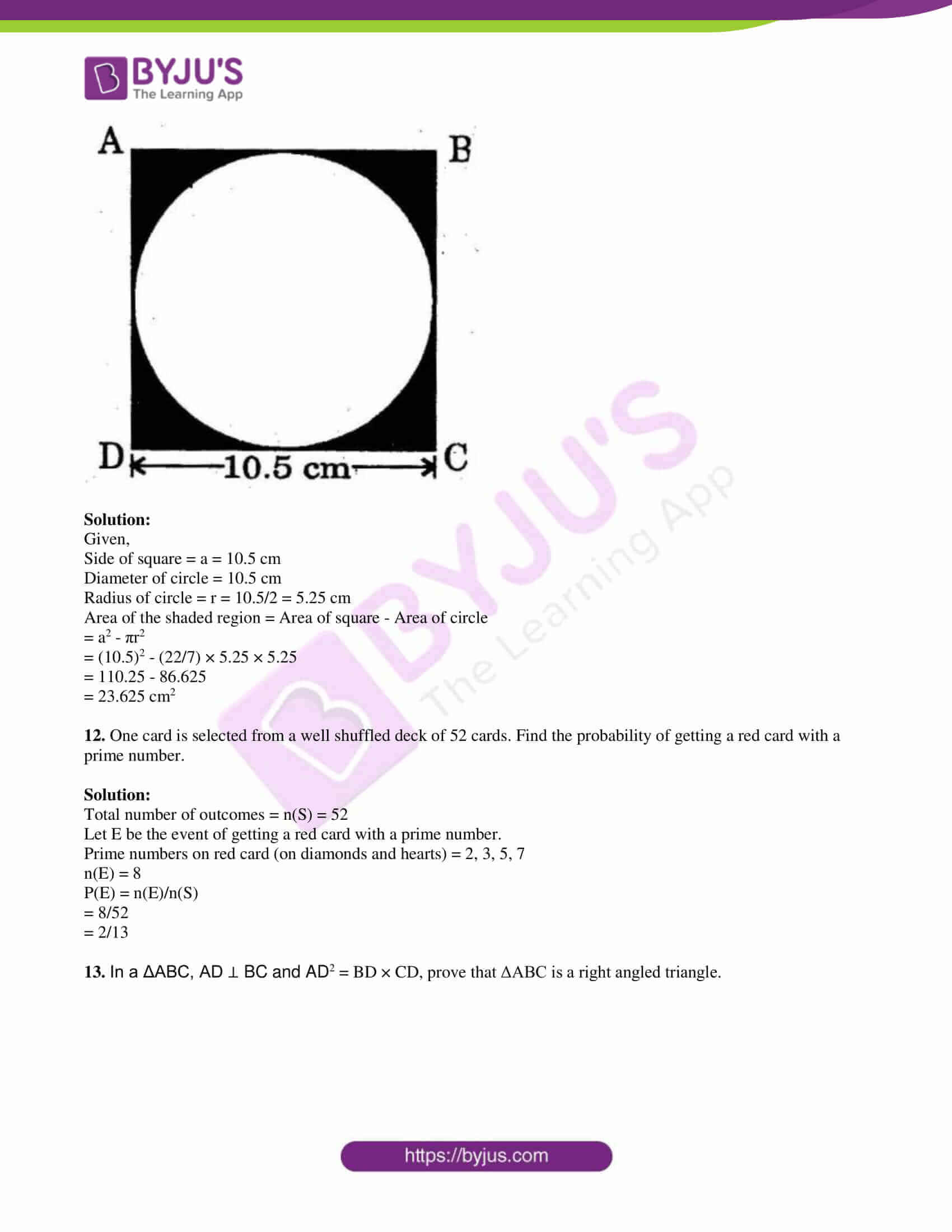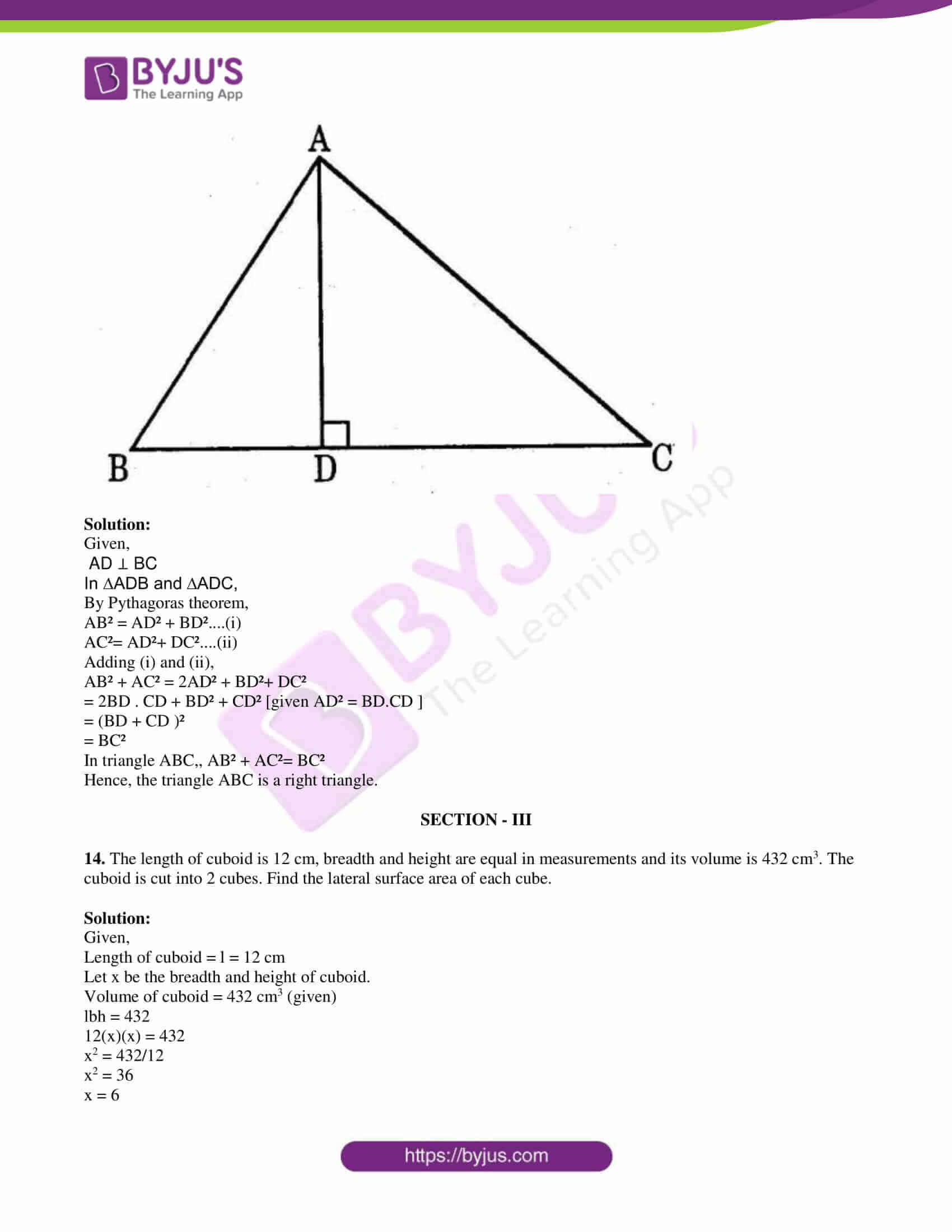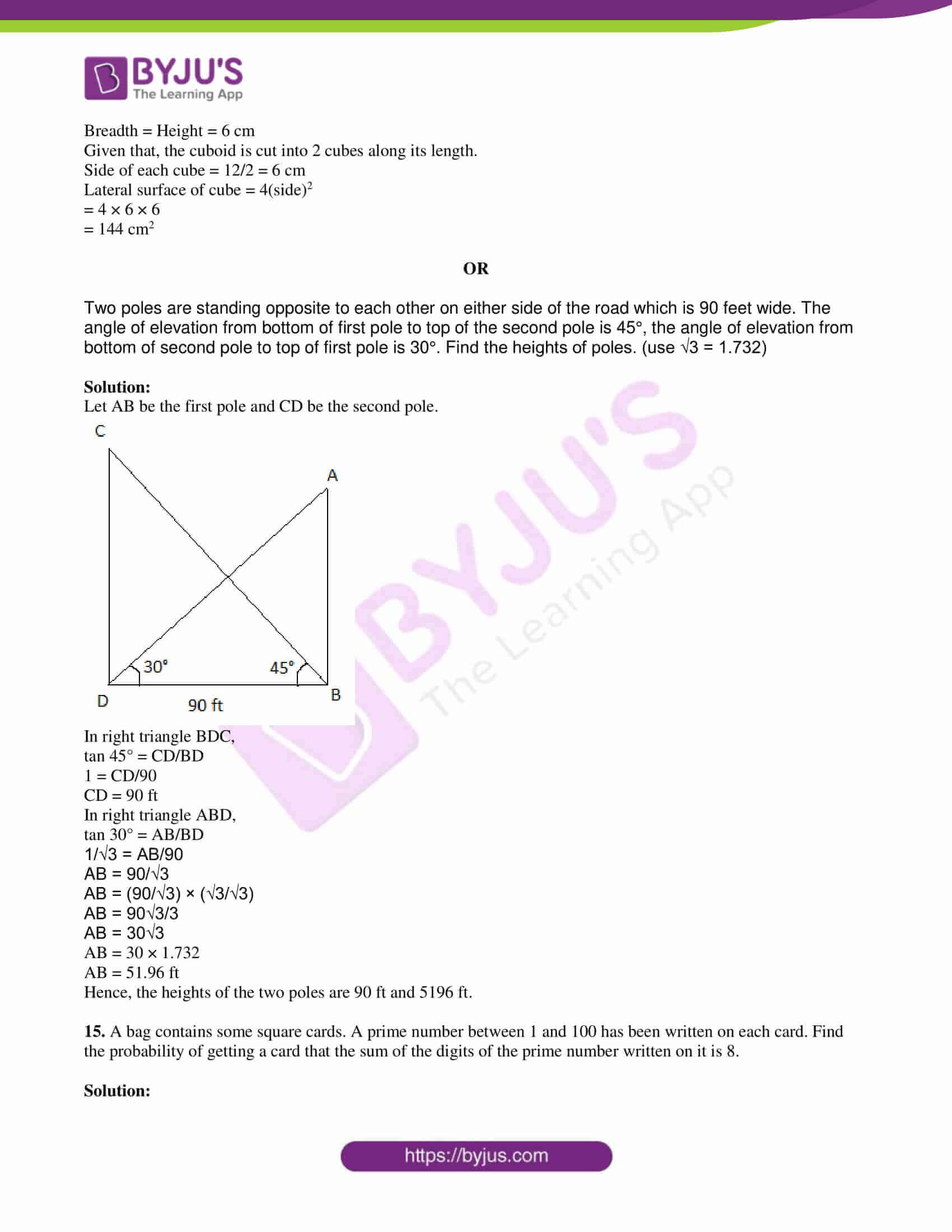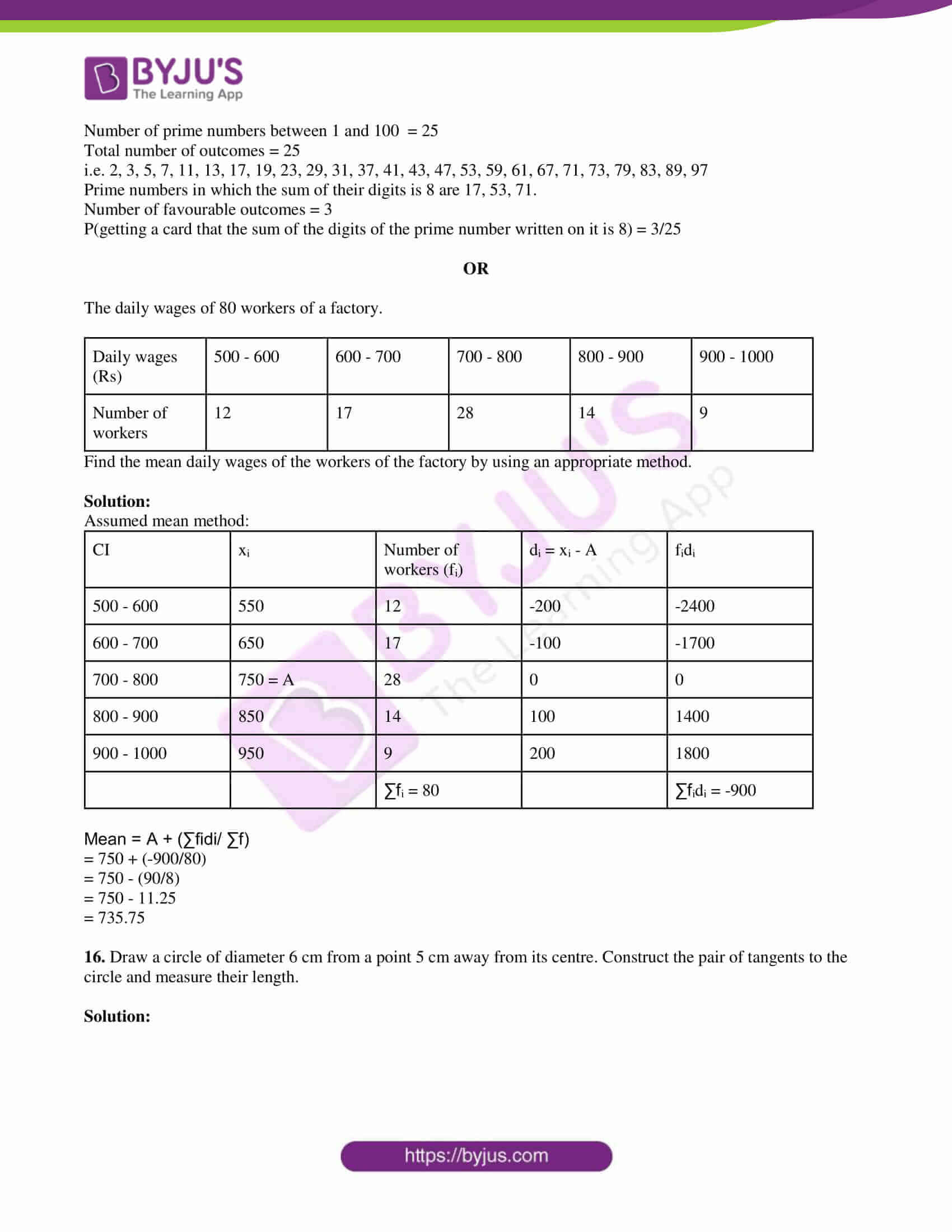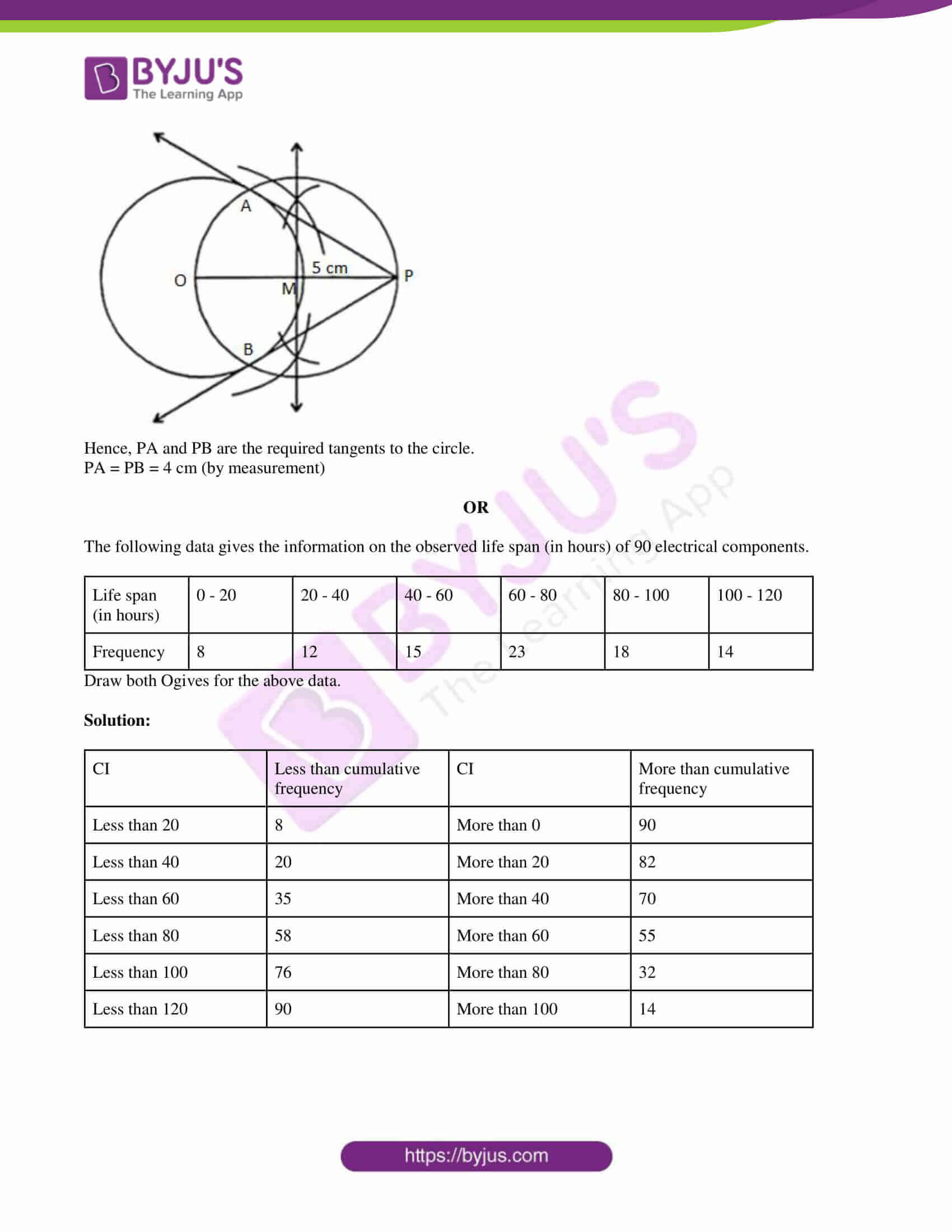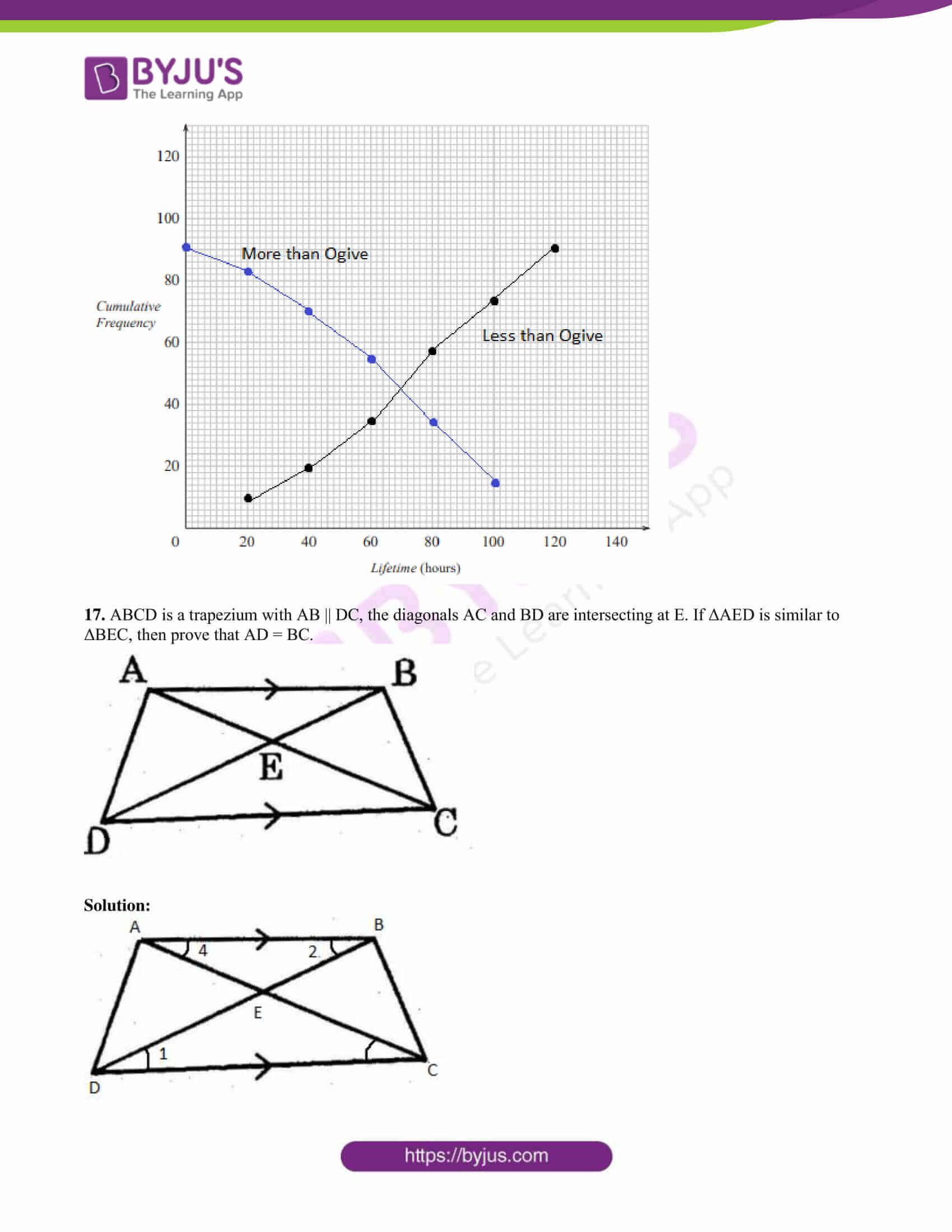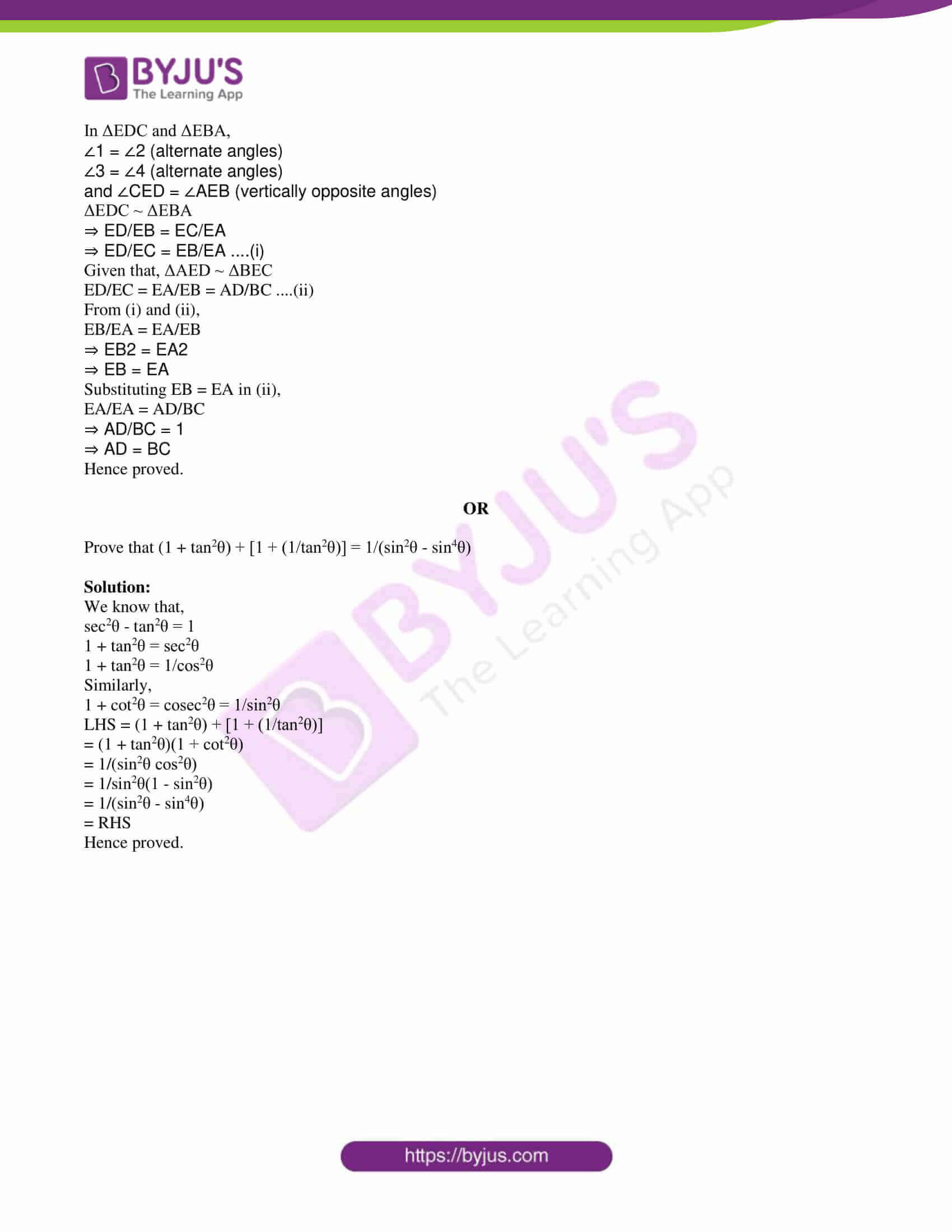PART A

SECTION – I

1. If sin A = 1/√2 and cot B = 1, prove that sin (A + B) = 1, where A and B both are acute angles.

Solution:

Given,

A and B are acute angles.

sin A = 1/√2

sin A = sin 45°

⇒ A = 45°

cot B = 1

cot B = cot 45°

⇒ B = 45°

Now,

sin (A + B) = sin (45° + 45°)

= sin 90°

= 1

Hence proved.

2. The length of the minute hand of a clock is 3.5 cm. Find the area swept by a minute hand in 30 minutes. (use π = 22/7)

Solution:

Given,

Length of a minute hand = 3.5 cm

The minute hand forms a semicircle in 30 min.

Thus, radius = r = 3.5 cm

Area = πr2/2

= (1/2) × (22/7) × 3.5 × 3.5

= 19.25

Hence, the area swept by the minute hand of a clock is 19.25 cm2.

3. Express cos θ in terms of tan θ.

Solution:

tan θ = sin θ/cos θ

Squaring on both sides,

tan2θ = sin2θ/cos2θ

tan2θ = (1 – cos2θ)/cos2θ

tan2θ = (1/cos2θ) – 1

tan2θ + 1 = 1/cos2θ

⇒ cos2θ = 1/(1 + tan2θ)

⇒ cos θ = 1/√(1 + tan2θ)

4. From the first 50 natural numbers, find the probability of a randomly selected number is a multiple of 3.

Solution:

Total number of outcomes = n(S) = 50

i.e. the first 50 natural numbers.

Let E be the event of getting a number which is multiple of 3.

E = {3, 6, 9, 12, 15, 18, 21, 24, 27, 30, 33, 36, 39, 42, 45, 48}

n(E) = 16

P(E) = n(E)/n(S)

= 16/50

= 8/25

Hence, the required probability is 8/25.

5. Write the formula to find the curved surface area of a cone and explain each term in it.

Solution:

Curved surface area of a cone = πrl

Here,

r = Radius of the circular base

l = Slant height

6. “The median of observations -2, 5, 3, -1, 4, 6 is 3.5”. Is it correct? Justify your answer.

Solution:

Given observations are:

-2, 5, 3, -1, 4, 6

Ascending order:

-2, -1, 3, 4, 5, 6

Number of observations = n = 6

Median = ½ [(n/2)th + {(n/2) + 1}th] observations

= ½ [3rd + 4th observations]

= ½ [3 + 4]

= 7/2

= 3.5

Therefore, the median is 3.5.

7. If cos θ = 1/√2, then find the value of 4 + cot θ.

Solution:

Given,

cos θ = 1/√2

cos θ = cos 45°

⇒ θ = 45°

4 + cot θ = 4 + cot 45°

= 4 + 1

= 5

SECTION – II

8. The diameter of a solid sphere is 6 cm. It is melted and recast into a solid cylinder of height 4 cm. Find the radius of the cylinder.

Solution:

Given,

Diameter of sphere = 6 cm

Radius of sphere = r = 6/2 = 3 cm

Height of cylinder = h = 4 cm

Let R be the radius of the cylinder.

Given that, the sphere is melted and recast into a cylinder.

Volume of sphere = Volume of cylinder

(4/3)πr3 = πR2h

(4/3) × (22/7) × 3 × 3 × 3 = (22/7) × R2 × 4

R2 = 36/4

R2 = 9

R = 3 cm

Hence, the radius of the cylinder is 3 cm.

9. Write the formula of mode for grouped data and explain each term in it.

Solution:

Mode for grouped data = l + [(f1 – f0)/ (2f1 – f0 – f2)] × h

Here,

l = Lower limit of the modal class

f1 = Frequency of the modal class

f0 = Frequency of the class preceding the modal class

f2 = Frequency of the class succeeding the modal class

h = class size (or class height)

10. A person 25 mts away from a cell tower observes the top of the cell tower at an angle of elevation 30°. Draw the suitable diagram for this situation.

Solution:

Let AB be the cell tower and C be the point of observation.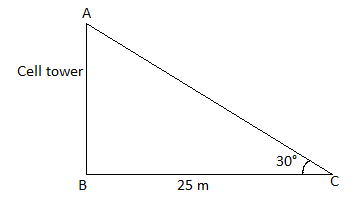11. Find the area of the shaded region in the given figure. ABCD is a square with side 10.5 cm.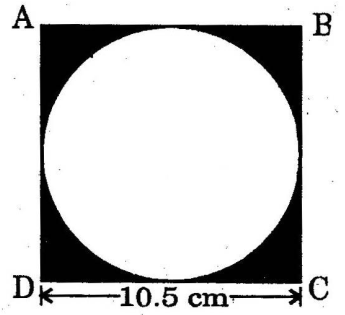Solution:

Given,

Side of square = a = 10.5 cm

Diameter of circle = 10.5 cm

Radius of circle = r = 10.5/2 = 5.25 cm

Area of the shaded region = Area of square – Area of circle

= a2 – πr2

= (10.5)2 – (22/7) × 5.25 × 5.25

= 110.25 – 86.625

= 23.625 cm2

12. One card is selected from a well shuffled deck of 52 cards. Find the probability of getting a red card with a prime number.

Solution:

Total number of outcomes = n(S) = 52

Let E be the event of getting a red card with a prime number.

Prime numbers on red card (on diamonds and hearts) = 2, 3, 5, 7

n(E) = 8

P(E) = n(E)/n(S)

= 8/52

= 2/13

13. In a ΔABC, AD ⊥ BC and AD2 = BD × CD, prove that ΔABC is a right angled triangle.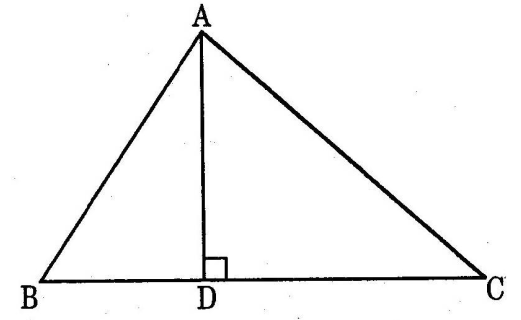Solution:

Given,

By Pythagoras theorem,

AB² + AC² = 2AD² + BD²+ DC²

= 2BD . CD + BD² + CD² [given AD² = BD.CD ]

= (BD + CD )²

= BC²

In triangle ABC,, AB² + AC²= BC²

Hence, the triangle ABC is a right triangle.

SECTION – III

14. The length of cuboid is 12 cm, breadth and height are equal in measurements and its volume is 432 cm3. The cuboid is cut into 2 cubes. Find the lateral surface area of each cube.

Solution:

Given,

Length of cuboid = l = 12 cm

Let x be the breadth and height of cuboid.

Volume of cuboid = 432 cm3 (given)

lbh = 432

12(x)(x) = 432

x2 = 432/12

x2 = 36

x = 6

Breadth = Height = 6 cm

Given that, the cuboid is cut into 2 cubes along its length.

Side of each cube = 12/2 = 6 cm

Lateral surface of cube = 4(side)2

= 4 × 6 × 6

= 144 cm2

OR

Two poles are standing opposite to each other on either side of the road which is 90 feet wide. The angle of elevation from bottom of first pole to top of the second pole is 45°, the angle of elevation from bottom of second pole to top of first pole is 30°. Find the heights of poles. (use √3 = 1.732)

Solution:

Let AB be the first pole and CD be the second pole.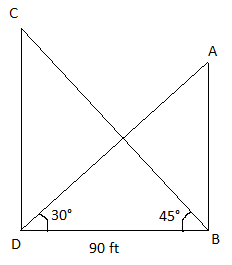In right triangle BDC,

tan 45° = CD/BD

1 = CD/90

CD = 90 ft

In right triangle ABD,

tan 30° = AB/BD

1/√3 = AB/90

AB = 90/√3

AB = (90/√3) × (√3/√3)

AB = 90√3/3

AB = 30√3

AB = 30 × 1.732

AB = 51.96 ft

Hence, the heights of the two poles are 90 ft and 5196 ft.

15. A bag contains some square cards. A prime number between 1 and 100 has been written on each card. Find the probability of getting a card that the sum of the digits of the prime number written on it is 8.

Solution:

Number of prime numbers between 1 and 100 = 25

Total number of outcomes = 25

i.e. 2, 3, 5, 7, 11, 13, 17, 19, 23, 29, 31, 37, 41, 43, 47, 53, 59, 61, 67, 71, 73, 79, 83, 89, 97

Prime numbers in which the sum of their digits is 8 are 17, 53, 71.

Number of favourable outcomes = 3

P(getting a card that the sum of the digits of the prime number written on it is 8) = 3/25

OR

The daily wages of 80 workers of a factory.

 Daily wages (Rs) 500 – 600 600 – 700 700 – 800 800 – 900 900 – 1000 Number of workers 12 17 28 14 9

Find the mean daily wages of the workers of the factory by using an appropriate method.

Solution:

Assumed mean method:

 CI xi Number of workers (fi) di = xi – A fidi 500 – 600 550 12 -200 -2400 600 – 700 650 17 -100 -1700 700 – 800 750 = A 28 0 0 800 – 900 850 14 100 1400 900 – 1000 950 9 200 1800 ∑fi = 80 ∑fidi = -900

Mean = A + (∑fidi/ ∑f)

= 750 + (-900/80)

= 750 – (90/8)

= 750 – 11.25

= 735.75

16. Draw a circle of diameter 6 cm from a point 5 cm away from its centre. Construct the pair of tangents to the circle and measure their length.

Solution: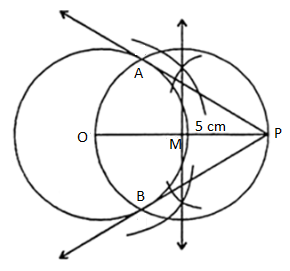Hence, PA and PB are the required tangents to the circle.

PA = PB = 4 cm (by measurement)

OR

The following data gives the information on the observed life span (in hours) of 90 electrical components.

 Life span (in hours) 0 – 20 20 – 40 40 – 60 60 – 80 80 – 100 100 – 120 Frequency 8 12 15 23 18 14

Draw both Ogives for the above data.

Solution:

 CI Less than cumulative frequency CI More than cumulative frequency Less than 20 8 More than 0 90 Less than 40 20 More than 20 82 Less than 60 35 More than 40 70 Less than 80 58 More than 60 55 Less than 100 76 More than 80 32 Less than 120 90 More than 100 1417. ABCD is a trapezium with AB || DC, the diagonals AC and BD are intersecting at E. If ΔAED is similar to ΔBEC, then prove that AD = BC.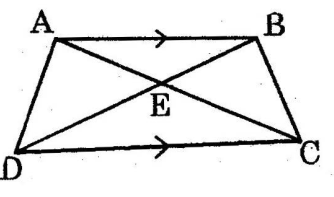Solution: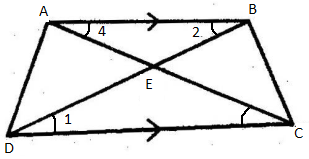In ΔEDC and ΔEBA,

∠1 = ∠2 (alternate angles)

∠3 = ∠4 (alternate angles)

and ∠CED = ∠AEB (vertically opposite angles)

ΔEDC ~ ΔEBA

⇒ ED/EB = EC/EA

⇒ ED/EC = EB/EA ….(i)

Given that, ΔAED ~ ΔBEC

ED/EC = EA/EB = AD/BC ….(ii)

From (i) and (ii),

EB/EA = EA/EB

⇒ EB2 = EA2

⇒ EB = EA

Substituting EB = EA in (ii),

Hence, proved.

OR

Prove that (1 + tan2θ) + [1 + (1/tan2θ)] = 1/(sin2θ – sin4θ)

Solution:

We know that,

sec2θ – tan2θ = 1

1 + tan2θ = sec2θ

1 + tan2θ = 1/cos2θ

Similarly,

1 + cot2θ = cosec2θ = 1/sin2θ

LHS = (1 + tan2θ) + [1 + (1/tan2θ)]

= (1 + tan2θ)(1 + cot2θ)

= 1/(sin2θ cos2θ)

= 1/sin2θ(1 – sin2θ)

= 1/(sin2θ – sin4θ)

= RHS

Hence, proved.